This article about Boeing 747 Altitude Dynamical Model SYSTEM DYNAMICS in an electronics circuit and experiment. Control inputs and motion vectors of an aircraft is shown in Figure 1.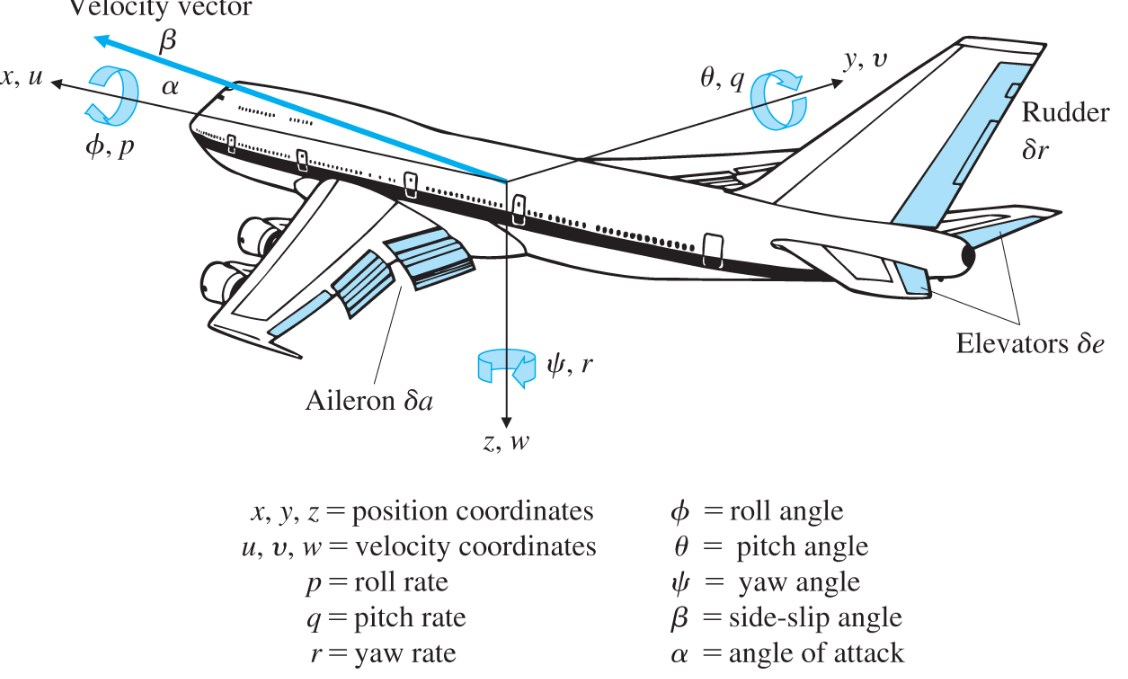The equations of motion for this system is

### Non – linear Model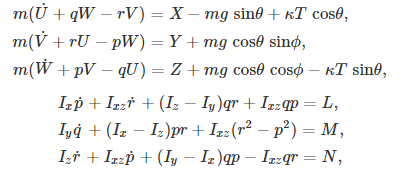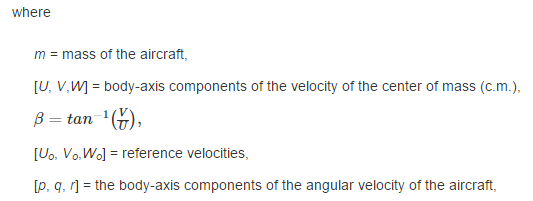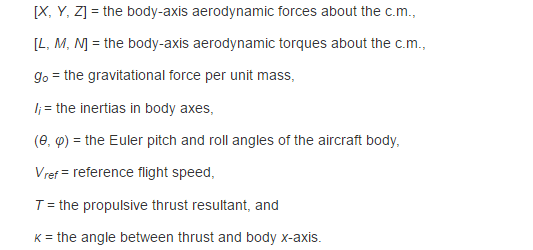### Linearized Model at Trim Condition

The equations of motion for this system is Linearized Model at Trim Condition At constant speed, level, straight flight condition equilibrium (trim) state space representation for altitude hold dynamics is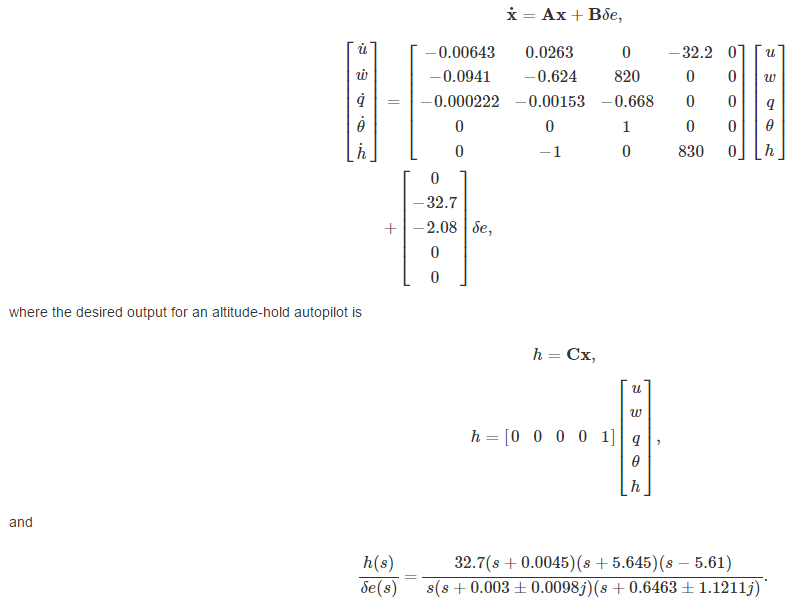## Project

1.  Implement an active analog electrical circuit using OPAMPs showing an equivalent dynamic behaviour to the altitude-hold model.
2.  Compare the model and the physical circuits simulations using the existing component values.
1.  Realize the the physically simulated circuit on a breadboard and compare the breadboardresponse to physical simulation

Deliverables, Submit

1.  Report showing your analysis, model, simulations, and actual device, and conclusions
2.  Black-box with one input ??(?) for elevator signal and one output h(?) terminals whose voltagerepresenting the plane altitude.

### Calculation of G1,G2,G3DC[]=DC[]=4803=let assume thatis equal to 500k ohm than,